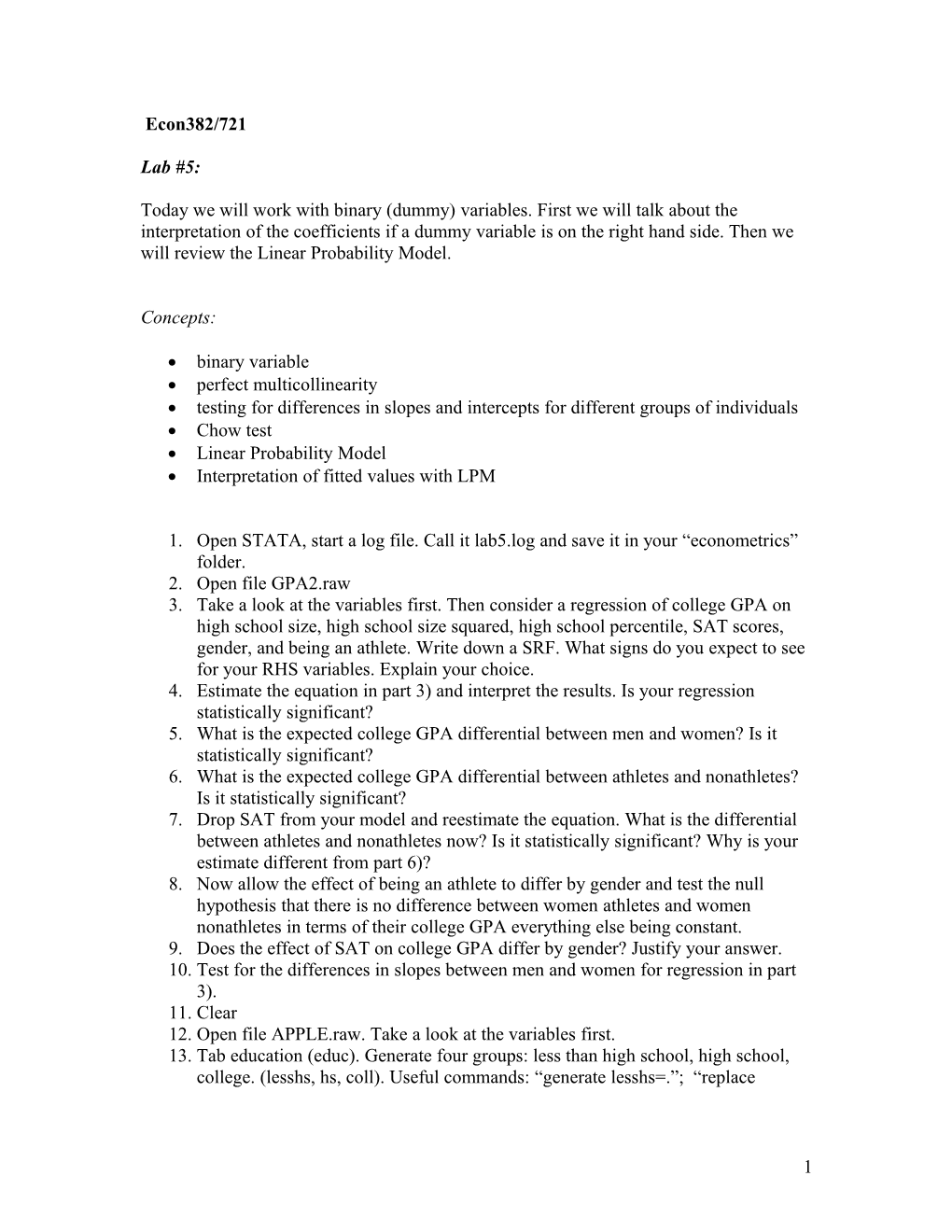Today We Will Work with Binary (Dummy) Variables. First We Will Talk About the InterpretationEcon382/721

Lab #5:

Today we will work with binary (dummy) variables. First we will talk about the interpretation of the coefficients if a dummy variable is on the right hand side. Then we will review the Linear Probability Model.

Concepts:

• binary variable
• perfect multicollinearity
• testing for differences in slopes and intercepts for different groups of individuals
• Chow test
• Linear Probability Model
• Interpretation of fitted values with LPM
1. Open STATA, start a log file. Call it lab5.log and save it in your “econometrics” folder.
2. Open file GPA2.raw
3. Take a look at the variables first. Then consider a regression of college GPA on high school size, high school size squared, high school percentile, SAT scores, gender, and being an athlete. Write down a SRF. What signs do you expect to see for your RHS variables. Explain your choice.
4. Estimate the equation in part 3) and interpret the results. Is your regression statistically significant?
5. What is the expected college GPA differential between men and women? Is it statistically significant?
6. What is the expected college GPA differential between athletes and nonathletes? Is it statistically significant?
7. Drop SAT from your model and reestimate the equation. What is the differential between athletes and nonathletes now? Is it statistically significant? Why is your estimate different from part 6)?
8. Now allow the effect of being an athlete to differ by gender and test the null hypothesis that there is no difference between women athletes and women nonathletes in terms of their college GPA everything else being constant.
9. Does the effect of SAT on college GPA differ by gender? Justify your answer.
10. Test for the differences in slopes between men and women for regression in part 3).
11. Clear
12. Open file APPLE.raw. Take a look at the variables first.
13. Tab education (educ). Generate four groups: less than high school, high school, college. (lesshs, hs, coll). Useful commands: “generate lesshs=.”; “replace lesshw=1 if educ<12”; “replace lesshw=0 if educ>=12”. Keep going for all four variables. Also check if all the four variables add up to 1.
14. Define a binary variable as ecobuy=1 is ecolbs>0 and ecobuy=0 if ecolbs=0. Ecobuy indicates whether, at the prices given, a family would buy any ecologically friendly apples. What fraction of the families claim they would buy ecolabeled apples?
15. Regress ecobuy on ecoprc regprc faminc hhsize edu age. Interpret all the coefficients.
16. Are the RHS variables jointly significant? Are they significant individually at 1, 5, or 10%?
17. Are the non-price variables jointly significant? Use the F-stat.
18. Which explanatory variable in part 13) seems to have the most important effect on the decision to buy ecolabeled apples?
19. In the estimation in part 13), how many estimated probabilities are negative or bigger than 100%? Use command “predict fittedprob, xb”.
20. Now rerun the regression in part 13), but instead of education include the categories that you had created in part 11) and compare the buying habits of high school dropouts to those of people who went to college. What do you observe? Is there any differential between the two? Is it statistically significant?

1